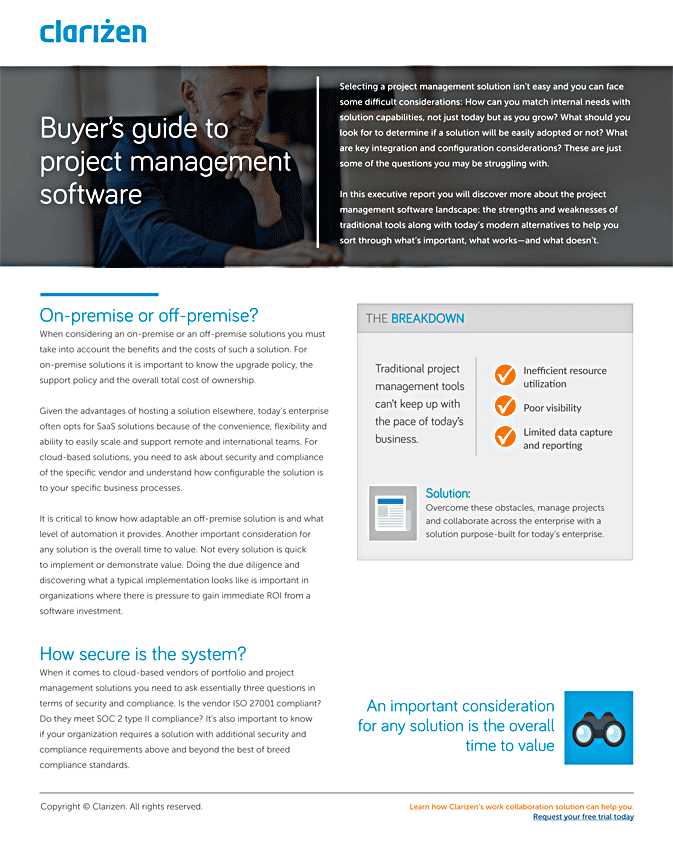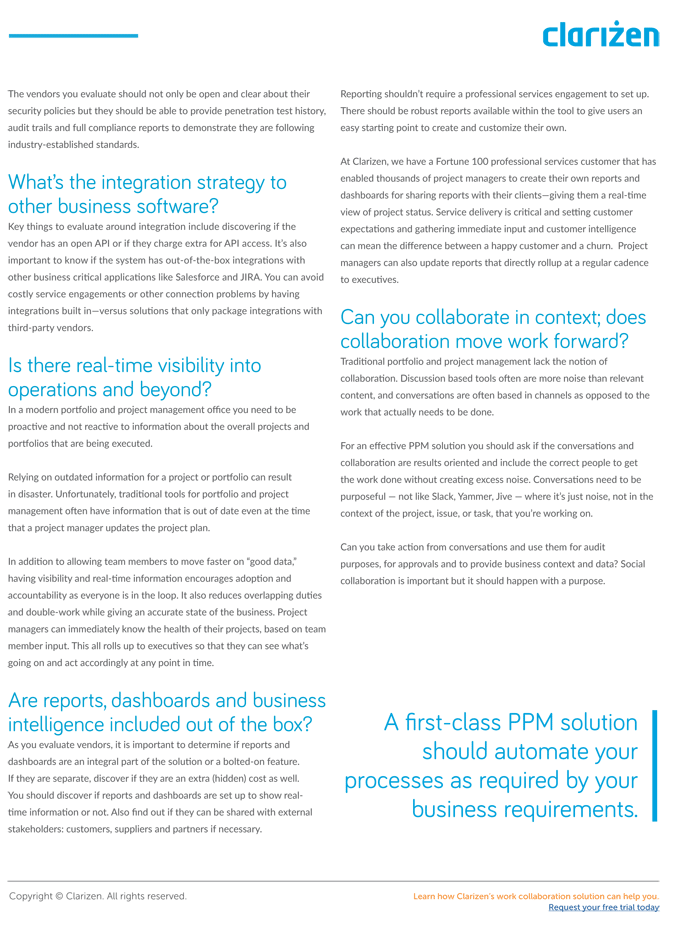← Go Back

Whether you are studying for project management certification, have had the role of project manager thrust upon you (funny how that happens, isn’t it?), or have been in the project management field for years and could use a refresher, here is a reference sheet that covers the basic financial terms and concepts on the project management landscape:Actual Cost (a.k.a. Actual Cost of Work Performed): The amount of money spent to date for an activity or work item based on actual labor and non-labor costs (e.g. materials, infrastructure, etc.).

Actual Revenue: The amount of actual revenue generated. This is calculated by multiplying time sheet duration by hourly billing rates, and adding both billable non-labor actual revenue and actual billed expenses.

Budgeted Amount: The total amount of funding allocated to a project. This is a top-down amount, and not time-phased.

Budgeted Cost (a.k.a. Budget at Completion): A rolled-up amount that is calculated by multiplying planned work by hourly cost rates, and then adding non-labor budget costs as applicable.

Budget Variance: The difference between the budgeted amount and the actual expenses incurred.

Cost Balance: The difference between the budgeted cost and the actual cost incurred.

Cost Performance Index: A ratio that measures the financial performance of a project. This is calculated by dividing the earned value by the actual cost of work performed. A CPI of higher than 1.0 indicates that the project is under budget.

Cost Variance: The difference between the earned value and the actual cost incurred.

Earned Value (a.k.a. Budgeted Cost of Work Performed): An indication of how much work has been completed over a specific time period. This value, which can be expressed in monetary terms or duration (e.g. hours), accrues during a project as individual work items are completed.

Estimate at Complete: A forecasted value of a project at completion. This is calculated by adding the actual cost and the estimate to complete.

Estimate to Complete: The expected amount of money required to complete the remainder of the project. This is calculated by subtracting the earned value from the budget cost, and dividing it by the cost performance index multiplied by the schedule performance index.

Expected Progress: A linear protection of the work involved in a specific task, which is distributed over that task’s duration as defined in the work plan. This metric is also dependent on the assigned resource or resources availability.

Expected Revenue: The forecasted revenue amount based on multiplying planned work by hourly billing rates, and adding billable non-labor budget revenue.

Planned Value (a.k.a. Budget at Completion): The authorized budget allocated to scheduled work items. This is calculated by multiplying expected progress by budgeted cost.

Remaining Budget: The amount of budget remaining in the project. This is calculated subtracting actual costs from budgeted amount.

Schedule Performance Index: A ratio that measures the schedule performance of a project. This is calculated by dividing the earned value by the planned value. An SPI of higher than 1.0 indicates that the project is ahead of schedule.

Choose Clarizen for Financial Planning Success

Clarizen’s robust built-in financial planning functionality combines the simplicity of a spreadsheet for data entry, with the granularity, control and structure that enterprises need to keep track of the bigger picture of project financial data at scale, and over time. To learn more, schedule your live guided demo, or launch your fully-fledged 30-day free trial of Clarizen today.## What are you waiting for?